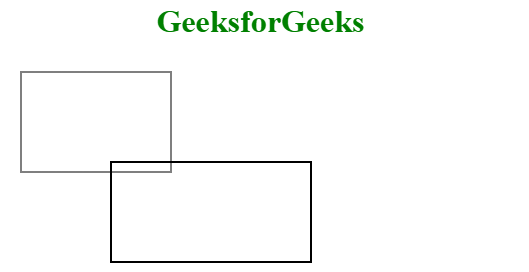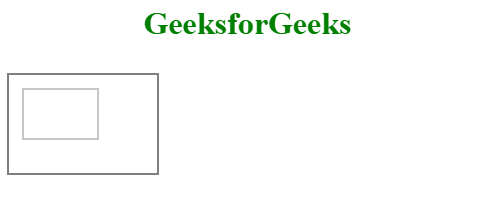# HTML | canvas scale() Method

The HTML canvas scale() Method is used to scale the current drawing into smaller or larger size. After scaling the drawing, all the feature of drawing scaled. It will have to define before the canvas.

Syntax:

`context.scale( scalewidth, scaleheight )`

Parameter Values:

• scalewidth: It scale the width of current drawing, (1=100%, 0.5=50%, 2=200%, and so on).
• scaleheight: It scale the height of current drawing, (1=100%, 0.5=50%, 2=200%, and so on)

Example 1: This example uses canvas scale() Method to increase the drawing size.

 `  ` `<``html``>  ` ` `  `<``head``>  ` `    ``<``title``>  ` `        ``HTML canvas scale() Method ` `    ``  ` `  ` ` `  `<``body``>  ` `<``center``> ` `<``h1` `style``=``"color:green"``>GeeksforGeeks ` `    ``<``canvas` `id``=``"GFG"` `            ``width``=``"500"` `            ``height``=``"300"``>  ` `  ` ` `  `    ``<``script``>  ` `        ``var x =  ` `            ``document.getElementById("GFG");  ` `        ``var contex =  ` `            ``x.getContext("2d");  ` `        ``contex.strokeRect(10, 10, 150, 100);  ` `        ``contex.scale(2, 2); ` `        ``contex.strokeRect(50, 50, 150, 100); ` `    ``  ` `<``center``> ` `  ` ` `  `  `

Output:Example 2: This example uses canvas scale() Method to decrease the drawing size.

 `  ` `<``html``>  ` ` `  `<``head``>  ` `    ``<``title``>  ` `        ``HTML canvas scale() Method ` `    ``  ` `  ` ` `  `<``body``>  ` `<``center``> ` `<``h1` `style``=``"color:green"``>GeeksforGeeks ` `    ``<``canvas` `id``=``"GFG"` `            ``width``=``"500"` `            ``height``=``"300"``>  ` `  ` ` `  `    ``<``script``>  ` `        ``var x = document.getElementById("GFG");  ` `        ``var contex = x.getContext("2d");  ` `        ``contex.strokeRect(10, 10, 150, 100);  ` `        ``contex.scale(0.5, 0.5); ` `        ``contex.strokeRect(50, 50, 150, 100); ` `    ``  ` ` ` `  ` ` `  `                     `

Output:Supported Browsers: The browser supported by HTML canvas scale() Method are listed below:

• Internet Explorer 9.0 or above
• Firefox
• Safari
• Opera

My Personal Notes arrow_drop_upCheck out this Author's contributed articles.

If you like GeeksforGeeks and would like to contribute, you can also write an article using contribute.geeksforgeeks.org or mail your article to contribute@geeksforgeeks.org. See your article appearing on the GeeksforGeeks main page and help other Geeks.

Please Improve this article if you find anything incorrect by clicking on the "Improve Article" button below.

Article Tags :
Practice Tags :

Be the First to upvote.

Please write to us at contribute@geeksforgeeks.org to report any issue with the above content.